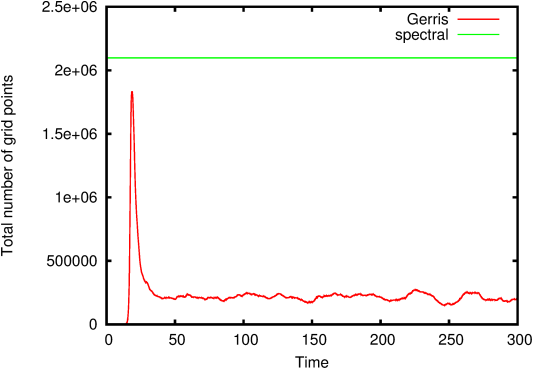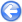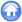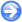### 3.5Forced isotropic turbulence in a triply-periodic box

Author
Kristjan Gudmundsson
Command
mpirun -np 8 gerris3D -m -s1 forcedturbulence.gfs
Version
110131
Required files
spectral.dat multiview.gfv
Running time
22 hours on 8 AMD Opteron 2GHz

We compute the evolution of forced isotropic turbulence (see ) and compare Gerris’ solution to that of the hit3d pseudo-spectral code. The initial condition is an unstable solution to the incompressible Euler equations. Numerical noise in the solution eventually leads to the destabilisation of the base solution into a fully turbulent flow where turbulent dissipation balances the linear input of energy (as illustrated graphically in the animation of Figure 26).

The two codes agree at early time, or until the solution transitions to a turbulent state. This happens earlier in Gerris as the symmetry of the base state is not preserved with the same accuracy as in the pseudo-spectral code (the main reason being the tolerance on non-divergence of the incompressible velocity field). Note however that the statistics produced by the two codes agree well after transition to turbulence.

Adaptivity is used in Gerris to reduce the computational cost. Figure 30 illustrates the number of grid points as a function of time.

 Figure 26: Animation of the evolution of the λ2 isosurface (a way to characterise vortices), cross-sections of the level of refinement (bottom plane), of the magnitude of vorticity (right plane) and pressure (left plane).

 Figure 27: Evolution of kinetic energy as computed via Gerris and a pseudo-spectral code. Note how the energy grows exponentially before the flow finally transitions to turbulence. This is because the laminar solution is relatively smooth and its dissipation is unable to balance the energy input.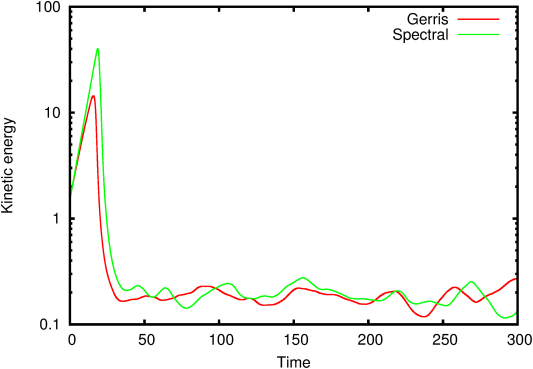Figure 28: The dissipation function. The dissipation increases exponentially during the laminar stage as it is proportional to energy at this stage. During transition the dissipation increases drastically as the flow gains energy at higher wavenumbers.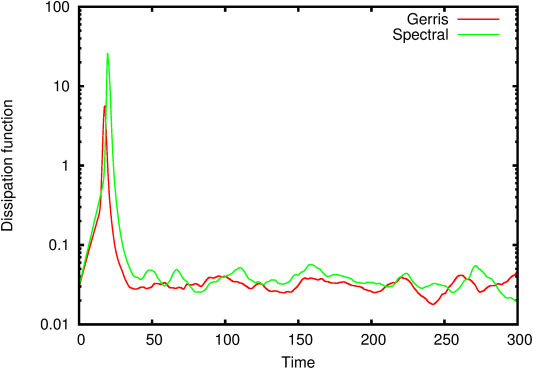Figure 29: The microscale Reynolds number.Figure 30: Number of grid points as a function of time for Gerris and the spectral code.